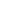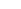# Kleene Plus Closure of Regular Grammars

## Algorithm

Given regular grammar G, we would like to construct a regular grammar G' such that L(G') = (L(G))+:

1. removefrom the language described by G (see the relevant section), thereby removing all-productions from the grammar;
2. for every rule in the form Aa (A being a non-terminal and a a single terminal), we add the rule AaS, where S is the start symbol of G;
3. if Swas originally in the productions of G, we addto the language generated by the grammar (see the relevant section)

## Example

Give a regular grammar recognising the Kleene plus closure of the language recognised by the following grammar:

 SaA | bB AaA | a |Bc | bS |where S is the start symbol.

1. Removefrom the language:

 Sa | b | aA | bB AaA | a Bc | bS

where S is the start symbol.

Note thatwas not in the language, but the procedure removes the undesirable-productions in the grammar.

2. For every rule in the form Aa (A being a non-terminal and a a single terminal), we add the rule AaS:

 Sa | aS | b | bS | aA | bB AaA | a | aS Bc | cS | bS

where S is the start symbol.

3. Since the language did not contain the empty string (since Swas not in the grammar), we are done.

## Exercises

Construct regular grammars which recognise the Kleene plus closure of the languages described using the following regular grammars:

1. G = < { a, b, c },
{ S, A, B, C },
 { SaA | bC, AaA | bB |, BbB | b |, CcB | cC |},
S >
2. G = < { a, b, c },
{ S, A, B, C },
 { SaA | bC |, AaA | aS, Bb, CcB | cS },
S >
3. G = < { a, b, c },
{ S, A, B, C },
 { SaA | bC |, AaA | aS, Bb, CcB | cS },
S >
4. G = < { a, b },
{ S, A },
 { SaA | bS, A},
S >
5.  G = < { a }, { S }, { S}, S >